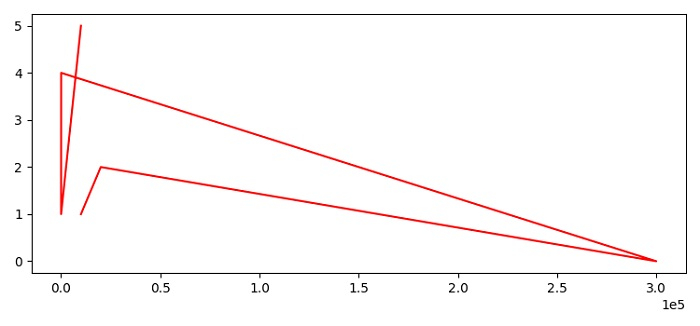# How to change the font size of scientific notation in Matplotlib?

To change the fontsize of scientific notation in matplotlib, we can take the following steps −

• Set the figure size and adjust the padding between and around the subplots.

• Make a list of x and y values.

• Plot x and y data points using plot() method.

• To change the font size of scientific notation, we can use style="sci" class by name.

• To display the figure, use show() method.

## Example

from matplotlib import pyplot as plt

plt.rcParams["figure.figsize"] = [7.50, 3.50]
plt.rcParams["figure.autolayout"] = True

x = [10000, 20000, 300000, 34, 1, 10000]
y = [1, 2, 0, 4, 1, 5]

plt.plot(x, y, color='red')
plt.ticklabel_format(axis="x", style="sci", scilimits=(0, 0))

plt.show()

## Output